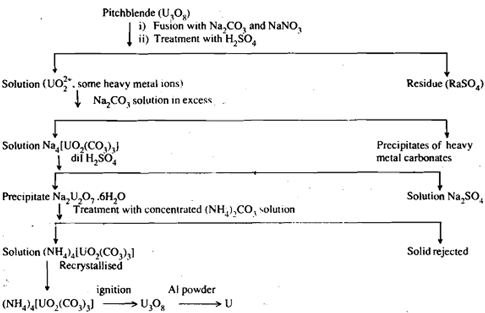## Show the chemical properties of uranium, Chemistry

Assignment Help:

Q. Show the chemical properties of Uranium?

Uranium is mainly extracted from pitchblende. The concentrated ore (pitchblende, U3O8) is washed and then fused with sodium nitrate and sodium carbonate. The fused pass in treated with sulphuric acid, which extracts uranyl sulphate, U02S04. Addition of sodium carbonate solution in excess to the above solution, removes all the heavy metals as carbonates. Uranium goes in solution as sodium uranyl carbonate Na4 [U02(C03)3]. Addition of dilute toH2SO4 the dradyl carbonate solution precipitates uranium as sodium diuranate, Na2U207which on treatment with concentrated solution of (NH4)2C03 passes into solution as ammonium uranyl carbonate, (NH4)4[U02 (C03)3]. Concentration of this solution gives pure U3Os. Reduction of U308 with aluminium powder produces uranium metal. All these steps - involved in extraction of uranium from pitchblende are summarised in a flow sheet.#### What are cell diagrams - galvanic cell, What are Cell diagrams? Conside...

What are Cell diagrams? Consider the galvanic cell depicted in Figure. This cell has a hydrogen electrode at the left and a silver-silver chloride electrode at the right. The h

#### Which of the following is always a whole number, Which of the following is ...

Which of the following is always a whole number : (1) Atomic weight             (2) Atomic radii     (3) Equivalent weight   (4) Atomic number Ans:  Atomic number

#### The covalency of nitrogen, The covalency of nitrogen in  HNO 3 is: (1...

The covalency of nitrogen in  HNO 3 is: (1) 0   (2) 3    (3) 4                               (4) 5 Ans: 5

#### Conductance, what is gas ion electrode?

what is gas ion electrode?

#### Mole concept, how much time will it require to spend 0.1 mole of rupees whe...

how much time will it require to spend 0.1 mole of rupees when the average rate of expenditure is 1 billion rupees per second?

#### Density, You are given a sample block of an unknown metal. The block disp...

You are given a sample block of an unknown metal. The block displaces 3.24 mL of water and has a mass of 62.5429 g. What is the density of the unknown metal? What is the metal?

#### Full Balanced Equations, #question.What is the balanced equation for the re...

#question.What is the balanced equation for the reaction of aluminium nitrate solution and sodium carbonate solution; where aluminium hydroxide is formed.

#### Calculate ionic products of water(kw) at a given temperature, It looks at h...

It looks at how the ionic product varies with temperature, and how that ... page, we shall calculate the concentration of hydroxonium ions present in pure water. Kw is called

#### Milkans oil drop method, how was the magnitude of electric charge concluded...

how was the magnitude of electric charge concluded?

#### Colloids, conclusion for assignment on topic applications of colloids

conclusion for assignment on topic applications of colloids